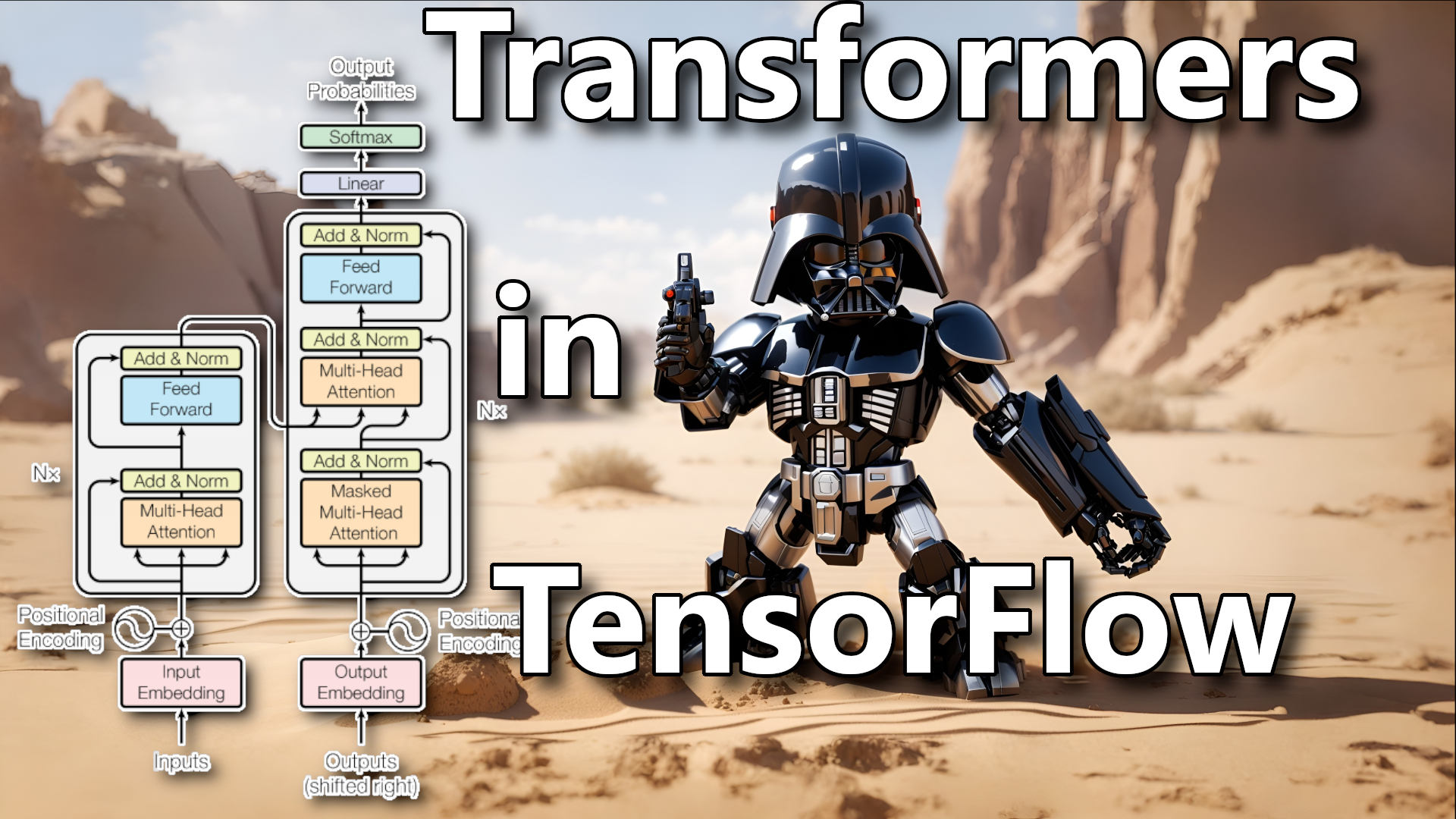# Building a Transformer model with Encoder and Decoder layers

## In this tutorial, we continue implementing the complete Transformer model in TensorFlow. To achieve this, we implement Encoder and Decoder step-by-step

In the first tutorial, we got familiar with Transformers. What are they, and what advantages and limitations do they bring. Also, we implemented the PositionalEmbedding layer in TensorFlow.

After the first tutorial, we moved to the second tutorial. In the second tutorial, we implemented `Add & Norm`, `BaseAttention`, `CrossAttention`, `GlobalSelfAttention`, `CausalSelfAttention`, and `FeedForward` layers.

So, using layers from the previous tutorials, we'll implement `Encoder` and `Decoder` layers that will be used to build a complete `Transformer` Model.

While implementing and testing content in this tutorial, I am not showing essential imports from the previous tutorial, so if you are testing everything by your self, follow the previous tutorial to import the necessary code.

## EncoderLayer layer

Let's start with the `EncoderLayer` layer. Why is it called EncoderLayer? Because it is a single layer of the `Encoder`. The `Encoder` is composed of multiple `EncoderLayers`. A similar structure we'll see in the `Decoder`: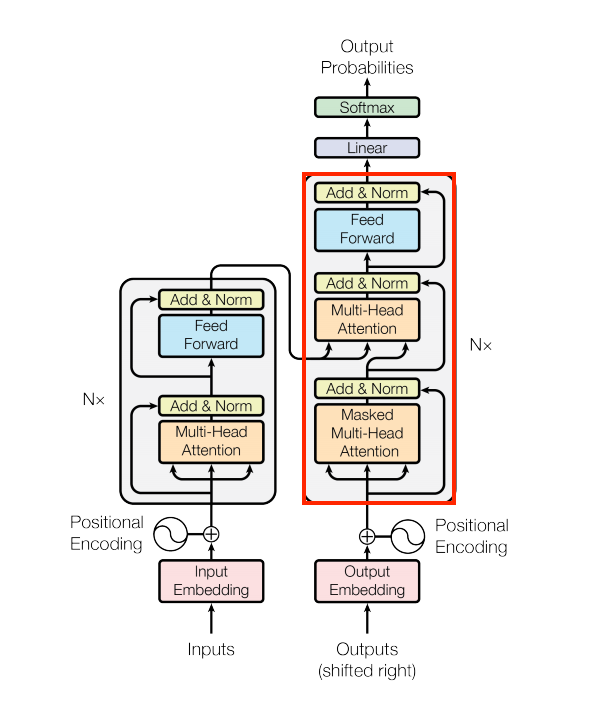The `EncoderLayer` consists of two sublayers: a `MultiHeadAttention` layer, more specifically `GlobalSelfAttention` layer, and a `FeedForward` layer. Each of these sublayers has a residual connection around it, followed by a layer normalization. Residual connections help to avoid the vanishing gradient problem in deep networks.

Let's implement this layer:

``````class EncoderLayer(tf.keras.layers.Layer):
"""
A single layer of the Encoder. Usually there are multiple layers stacked on top of each other.

Methods:
call: Performs the forward pass of the layer.

Attributes:
self_attention (GlobalSelfAttention): The global self-attention layer.
ffn (FeedForward): The feed-forward layer.
"""
def __init__(self, d_model: int, num_heads: int, dff: int, dropout_rate: float=0.1):
"""
Constructor of the EncoderLayer.

Args:
d_model (int): The dimensionality of the model.
dff (int): The dimensionality of the feed-forward layer.
dropout_rate (float): The dropout rate.
"""
super().__init__()

self.self_attention = GlobalSelfAttention(
key_dim=d_model,
dropout=dropout_rate
)

self.ffn = FeedForward(d_model, dff)

def call(self, x: tf.Tensor) -> tf.Tensor:
"""
The call function that performs the forward pass of the layer.

Args:
x (tf.Tensor): The input sequence of shape (batch_size, seq_length, d_model).

Returns:
tf.Tensor: The output sequence of shape (batch_size, seq_length, d_model).
"""
x = self.self_attention(x)
x = self.ffn(x)
return x``````

Now let's test it out. We will use the same random input as in the previous tutorial. The output shape should be the same as the input shape:

``````encoder_vocab_size = 1000
d_model = 512

encoder_embedding_layer = PositionalEmbedding(vocab_size, d_model)

random_encoder_input = np.random.randint(0, encoder_vocab_size, size=(1, 100))

encoder_embeddings = encoder_embedding_layer(random_encoder_input)

print("encoder_embeddings shape", encoder_embeddings.shape)

encoder_layer_output = encoder_layer(encoder_embeddings)

print("encoder_layer_output shape", encoder_layer_output.shape)``````

We'll see the following output:

``````encoder_embeddings shape (1, 100, 512)
encoder_layer_output shape (1, 100, 512)``````

Great! We have implemented the `EncoderLayer` layer. The output shape is the same as the input shape. This is because the output of the `EncoderLayer` is the same as the output of the `FeedForward` layer, which has the same shape as the input. Now, let's combine multiple `EncoderLayers` to create the `Encoder` layer.

## Encoder layer

Now let's implement the `Encoder` layer. The `Encoder` has integrated the `PositionalEmbedding` layer, the multiple of `EncoderLayer` layers, and the `Dropout` layer. The output of each `EncoderLayer` is passed to the next `EncoderLayer`. The output of the last `EncoderLayer` will be the output of the `Encoder`. In the following image, you can see the `Encoder` marked in red: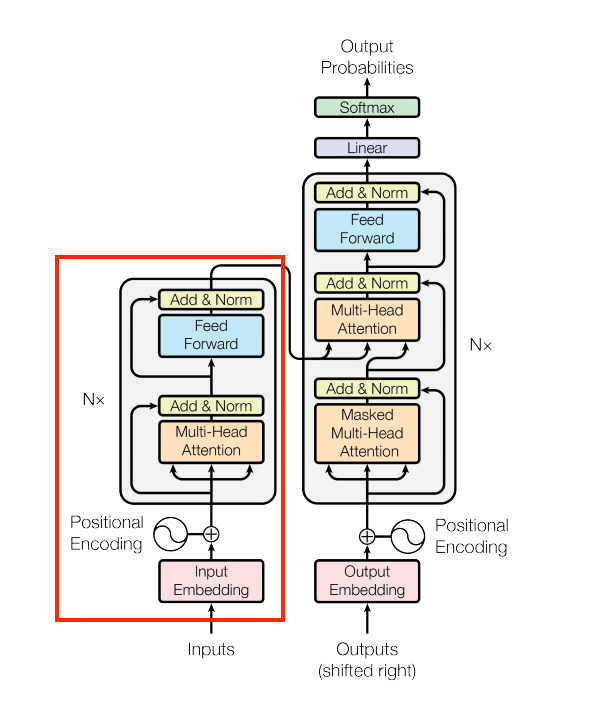The `Nx` represents the count of how many `EncoderLayers` we use in the whole `Encoder`. Let's implement the `Encoder` layer in the code:

``````class Encoder(tf.keras.layers.Layer):
"""
A custom TensorFlow layer that implements the Encoder. This layer is mostly used in the Transformer models
for natural language processing tasks, such as machine translation, text summarization or text classification.

Methods:
call: Performs the forward pass of the layer.

Attributes:
d_model (int): The dimensionality of the model.
num_layers (int): The number of layers in the encoder.
pos_embedding (PositionalEmbedding): The positional embedding layer.
enc_layers (list): The list of encoder layers.
dropout (tf.keras.layers.Dropout): The dropout layer.
"""
def __init__(self, num_layers: int, d_model: int, num_heads: int, dff: int, vocab_size: int, dropout_rate: float=0.1):
"""
Constructor of the Encoder.

Args:
num_layers (int): The number of layers in the encoder.
d_model (int): The dimensionality of the model.
dff (int): The dimensionality of the feed-forward layer.
vocab_size (int): The size of the vocabulary.
dropout_rate (float): The dropout rate.
"""
super().__init__()

self.d_model = d_model
self.num_layers = num_layers

self.pos_embedding = PositionalEmbedding(vocab_size=vocab_size, d_model=d_model)

self.enc_layers = [
EncoderLayer(d_model=d_model,
dff=dff,
dropout_rate=dropout_rate)
for _ in range(num_layers)]
self.dropout = tf.keras.layers.Dropout(dropout_rate)

def call(self, x: tf.Tensor) -> tf.Tensor:
"""
The call function that performs the forward pass of the layer.

Args:
x (tf.Tensor): The input sequence of shape (batch_size, seq_length).

Returns:
tf.Tensor: The output sequence of shape (batch_size, seq_length, d_model).
"""
x = self.pos_embedding(x)
# here x has shape `(batch_size, seq_len, d_model)`

x = self.dropout(x)

for i in range(self.num_layers):
x = self.enc_layers[i](x)

return x  # Shape `(batch_size, seq_len, d_model)`.``````

Following this and previous tutorials step-by-step, you should already see that we combined everything we covered before and constructed the `Encoder` layer. Let's test it out. We will use the same random input as before. As input, we'll generate a random sequence as before, which would be the sequence of tokenized words in real life.

``````encoder_vocab_size = 1000
d_model = 512

encoder = Encoder(num_layers=2, d_model=d_model, num_heads=2, dff=2048, vocab_size=encoder_vocab_size)

random_encoder_input = np.random.randint(0, encoder_vocab_size, size=(1, 100))

encoder_output = encoder(random_encoder_input)

print("random_encoder_input shape", random_encoder_input.shape)
print("encoder_output shape", encoder_output.shape)``````

You should see the following output:

``````random_encoder_input shape (1, 100)
encoder_output shape (1, 100, 512)``````

Now we have completely implemented the `Encoder` layer. If everything is clear up to this point, you can move on to the decoder part. But if you still need clarification, go back and reread the previous sections.

## DecoderLayer layer

The `DecoderLayer` is similar to the `EncoderLayer`, but it has an additional `CrossAttention` layer between the `CausalSelfAttention` layer and the `FeedForward` layer:The `CrossAttention` layer calculates the attention weights between the decoder input and the encoder output. The `CausalSelfAttention` layer calculates the attention weights between the decoder input and the decoder output. The `FeedForward` layer transforms the representation of the input sequence into a more suitable form for the task at hand.

Let's implement the `DecoderLayer` layer:

``````class DecoderLayer(tf.keras.layers.Layer):
"""
A single layer of the Decoder. Usually there are multiple layers stacked on top of each other.

Methods:
call: Performs the forward pass of the layer.

Attributes:
causal_self_attention (CausalSelfAttention): The causal self-attention layer.
cross_attention (CrossAttention): The cross-attention layer.
ffn (FeedForward): The feed-forward layer.
"""
def __init__(self, d_model: int, num_heads: int, dff: int, dropout_rate: float=0.1):
"""
Constructor of the DecoderLayer.

Args:
d_model (int): The dimensionality of the model.
dff (int): The dimensionality of the feed-forward layer.
dropout_rate (float): The dropout rate.
"""
super(DecoderLayer, self).__init__()

self.causal_self_attention = CausalSelfAttention(
key_dim=d_model,
dropout=dropout_rate)

self.cross_attention = CrossAttention(
key_dim=d_model,
dropout=dropout_rate)

self.ffn = FeedForward(d_model, dff)

def call(self, x: tf.Tensor, context: tf.Tensor) -> tf.Tensor:
"""
The call function that performs the forward pass of the layer.

Args:
x (tf.Tensor): The input sequence of shape (batch_size, seq_length, d_model). x is usually the output of the previous decoder layer.
context (tf.Tensor): The context sequence of shape (batch_size, seq_length, d_model). Context is usually the output of the encoder.
"""
x = self.causal_self_attention(x=x)
x = self.cross_attention(x=x, context=context)

# Cache the last attention scores for plotting later
self.last_attn_scores = self.cross_attention.last_attn_scores

x = self.ffn(x)  # Shape `(batch_size, seq_len, d_model)`.
return x``````

Let's do a short analysis of what we have done here. We have implemented the `DecoderLayer` layer. The `DecoderLayer` layer consists of three sublayers: a `CausalSelfAttention` layer, a `CrossAttention` layer, and a `FeedForward` layer. Each of these sublayers has a residual connection around it, followed by a layer normalization. The output of each sublayer is `LayerNormalization(x + Sublayer(x))`. The output of the `DecoderLayer` is the same as the output of the `FeedForward` layer, which has the same shape as the input.

If we take, for example, the translation task from Spanish to English, here `context` would be a Spanish sentence, and `x` would be an English sentence. At the first iteration, we don't have any input to the decoder, except some `<start>` token, and we have a complete sentence for the encoder. So we input these to this layer. Then it calculates the attention weights between the decoder input and the encoder output. Then it calculates the attention weights between the decoder input and the decoder output. Then it transforms the representation of the input sequence into a more suitable form for the task at hand. Then it outputs the result.

After the first iteration, we now have, for example, `<start> Hello` as output from the decoder. So we repeat the above steps until the end of the sentence. After all iterations, we have translated the sentence, for example, `<start> Hello, how are you? <end>`. That's the whole idea of iterations in the decoder.

As before, we need to test this layer. We'll generate a random integers list, which will be our tokenized sentence. Then we'll push this data into an embedding layer to give us embeddings for each token. Then we'll push this data into the `decoderLayer` layer along with the encoder output (we received while testing the encoder layer) and get the output. Let's do it:

``````# Test DecoderLayer layer
decoder_vocab_size = 1000
d_model = 512
dff = 2048

random_decoderLayer_input = np.random.randint(0, decoder_vocab_size, size=(1, 110))

decoder_embeddings = encoder_embedding_layer(random_decoderLayer_input)

decoderLayer_output = decoder_layer(decoder_embeddings, encoder_output)

print("random_decoder_input shape", random_decoderLayer_input.shape)
print("decoder_embeddings shape", decoder_embeddings.shape)
print("decoder_output shape", decoderLayer_output.shape)``````

You should see the following output:

``````random_decoder_input shape (1, 110)
decoder_embeddings shape (1, 110, 512)
decoder_output shape (1, 110, 512)``````

Great, it works as expected. Our `decoderLayer` output shape is the same as the embedding shape, meaning we can stack whatever layers count we want sequentially.

## Decoder layer

Now let's implement the `Decoder` layer, which is very similar to the `Encoder` layer. The `Decoder` has integrated the `PositionalEmbedding` layer, the multiple of `DecoderLayer` layers, and the `Dropout` layer. The output of each `DecoderLayer` is passed to the next `DecoderLayer`. The output of the last `DecoderLayer` is the output of the `Decoder`. In the following image, you can see the `Decoder` marked in red: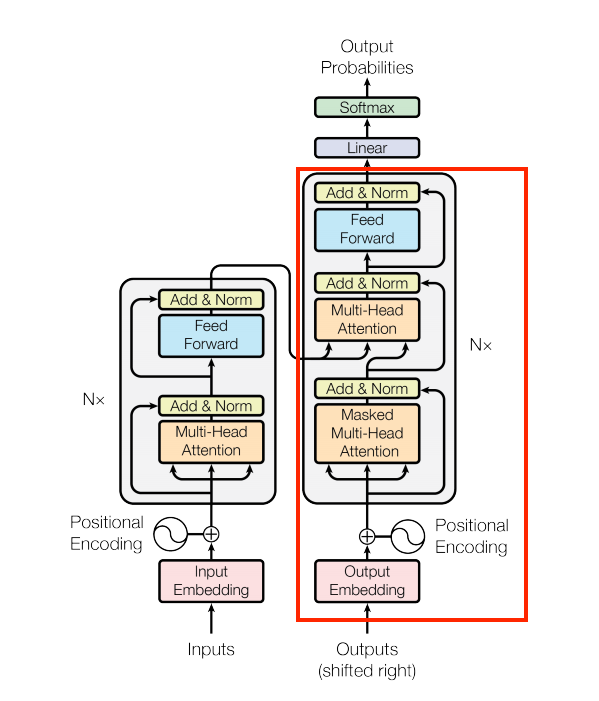Let's implement the `Decoder` layer in the code:

``````class Decoder(tf.keras.layers.Layer):
"""
A custom TensorFlow layer that implements the Decoder. This layer is mostly used in the Transformer models
for natural language processing tasks, such as machine translation, text summarization or text classification.

Methods:
call: Performs the forward pass of the layer.

Attributes:
d_model (int): The dimensionality of the model.
num_layers (int): The number of layers in the decoder.
pos_embedding (PositionalEmbedding): The positional embedding layer.
dec_layers (list): The list of decoder layers.
dropout (tf.keras.layers.Dropout): The dropout layer.
"""
def __init__(self, num_layers: int, d_model: int, num_heads: int, dff: int, vocab_size: int, dropout_rate: float=0.1):
"""
Constructor of the Decoder.

Args:
num_layers (int): The number of layers in the decoder.
d_model (int): The dimensionality of the model.
dff (int): The dimensionality of the feed-forward layer.
vocab_size (int): The size of the vocabulary.
dropout_rate (float): The dropout rate.
"""
super(Decoder, self).__init__()

self.d_model = d_model
self.num_layers = num_layers

self.pos_embedding = PositionalEmbedding(vocab_size=vocab_size, d_model=d_model)
self.dropout = tf.keras.layers.Dropout(dropout_rate)
self.dec_layers = [
DecoderLayer(
d_model=d_model,
dff=dff,
dropout_rate=dropout_rate) for _ in range(num_layers)]

self.last_attn_scores = None

def call(self, x: tf.Tensor, context: tf.Tensor) -> tf.Tensor:
"""
The call function that performs the forward pass of the layer.

Args:
x (tf.Tensor): The input sequence of shape (batch_size, target_seq_len).
context (tf.Tensor): The context sequence of shape (batch_size, input_seq_len, d_model).
"""
# `x` is token-IDs shape (batch, target_seq_len)
x = self.pos_embedding(x)  # (batch_size, target_seq_len, d_model)

x = self.dropout(x)

for i in range(self.num_layers):
x  = self.dec_layers[i](x, context)

self.last_attn_scores = self.dec_layers[-1].last_attn_scores

# The shape of x is (batch_size, target_seq_len, d_model).
return x``````

The `Decoder` class requires two inputs: a token-ID sequence representing the target sequence and an encoded input sequence, also known as the context. The decoder layer has multiple `DecoderLayer` instances that perform various operations on the input sequence to produce an output sequence.

When the `Decoder` object is instantiated, it sets up several layers, such as the `PositionalEmbedding` layer, responsible for adding positional information to the input token IDs, a `dropout` layer for regularization, and a stack of `DecoderLayer` instances.

The input token IDs go through the positional embedding and dropout layers during a forward pass. Then, for each `DecoderLayer`, the input undergoes causal self-attention, cross-attention, and a feed-forward neural network layer. The output of the last `DecoderLayer` is returned as the final output of the `Decoder`.

The `last_attn_scores` attribute of the `Decoder` instance stores the attention scores from the last decoder layer, which can be valuable for visualizing and debugging purposes.

Now, let's write a simple code to test the `Decoder` layer. We will use the same random input as before. As input, we'll generate a random sequence as before, what in real life would be the sequence of tokenized words:

``````# Test decoder layer
decoder_vocab_size = 1000
d_model = 512

decoder_layer = Decoder(num_layers=2, d_model=d_model, num_heads=2, dff=2048, vocab_size=decoder_vocab_size)

random_decoder_input = np.random.randint(0, decoder_vocab_size, size=(1, 100))

decoder_output = decoder_layer(random_decoder_input, encoder_output)

print("random_decoder_input shape", random_decoder_input.shape)
print("decoder_output shape", decoder_output.shape)``````

You should see the following output:

``````random_decoder_input shape (1, 100)
decoder_output shape (1, 100, 512)``````

Now we tested it with random data. But imagine, if it were actual data, then we would have, for example, a Spanish sentence as input and an English sentence as output. Then we would have to translate Spanish sentences to English sentences. We would have to input a Spanish sentence to the encoder and an English sentence to the decoder. Then we would have to iterate over the decoder until we get the `<end>` token. Then we would have translated sentences.

As we can see, the output decoder shape is a `(1, 100, 512)` vector. On this layer, we would have to apply the `argmax` function to get the most probable token and pick the word from the dictionary to get the final word. But we will do it later.

## The Transformer

Finally, we have implemented all the layers we need to build the `Transformer`. The `Transformer` consists of an `Encoder`, a `Decoder`, and a final linear layer. The output of the `Decoder` is the input to the final linear layer, and its result is returned as the output of the `Transformer`. The final `Dense` layer converts the resulting sequence into a probability distribution over the output vocabulary.

In the following image, you can see the `Transformer` model that we will implement: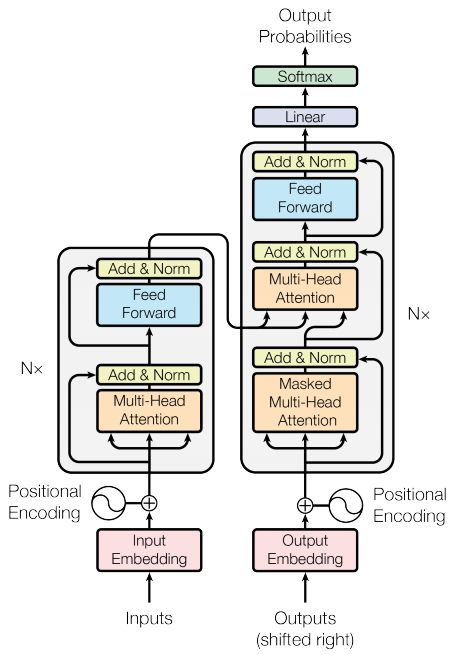Now let's implement the `Transformer` model in TensorFlow:

``````def Transformer(
input_vocab_size: int,
target_vocab_size: int,
encoder_input_size: int = None,
decoder_input_size: int = None,
num_layers: int=6,
d_model: int=512,
dff: int=2048,
dropout_rate: float=0.1,
) -> tf.keras.Model:
"""
A custom TensorFlow model that implements the Transformer architecture.

Args:
input_vocab_size (int): The size of the input vocabulary.
target_vocab_size (int): The size of the target vocabulary.
encoder_input_size (int): The size of the encoder input sequence.
decoder_input_size (int): The size of the decoder input sequence.
num_layers (int): The number of layers in the encoder and decoder.
d_model (int): The dimensionality of the model.
dff (int): The dimensionality of the feed-forward layer.
dropout_rate (float): The dropout rate.

Returns:
A TensorFlow Keras model.
"""
inputs = [
tf.keras.layers.Input(shape=(encoder_input_size,), dtype=tf.int64),
tf.keras.layers.Input(shape=(decoder_input_size,), dtype=tf.int64)
]

encoder_input, decoder_input = inputs

output = tf.keras.layers.Dense(target_vocab_size)(decoder)

return tf.keras.Model(inputs=inputs, outputs=output)``````

The `Transformer` incorporates both the `Encoder` and `Decoder` components to implement the `Transformer` architecture.

The `Encoder` is an instance of the `Encoder` class, responsible for taking a sequence of tokens as input and producing a sequence of contextual vectors, each representing information about a specific token in the input sequence.

The `Decoder` is also an instance of the `Decoder` class, which takes both a sequence of target tokens and the contextual information generated by the `Encoder` as input. It then generates a sequence of contextual vectors corresponding to each target token in the output sequence.

The `final_layer` is a Dense layer used to take the output from the `Decoder` and map it to a sequence of probabilities for the target tokens.

When we have a constructed `Transformer` Model, we provide an input tensor called `inputs`. This `inputs` tensor is actually a tuple containing two tensors: the `context` tensor (representing the input sequence for the Encoder) and the `x` tensor (representing the target sequence for the `Decoder`). When we call the `Transformer` model, it processes the context tensor through the `Encoder` to obtain contextual information for each token in the input sequence. It then uses this information and the `x` tensor to generate the output sequence through the `Decoder`. Finally, the model passes the output of the `Decoder` through the `final_layer` to obtain probabilities for the target tokens. The model returns both the logits (target token probabilities) and the attention weights.

To make this example more efficient, we reduced the size of layers, embeddings, and the internal dimensions of the `FeedForward` layer in the `Transformer` model. The original Transformer paper used a base model with `num_layers=6`, `d_model=512`,` num_heads=8`, and `dff=2048`. However, for testing purposes, we reduced these numbers.

``````encoder_input_size = 100
decoder_input_size = 110

encoder_vocab_size = 1000
decoder_vocab_size = 1000

model = Transformer(
input_vocab_size=encoder_vocab_size,
target_vocab_size=decoder_vocab_size,
encoder_input_size=encoder_input_size,
decoder_input_size=decoder_input_size,
num_layers=2,
d_model=512,
dff=512,
dropout_rate=0.1)

model.summary()``````

In the output, it should print the summary of the model:

``````__________________________________________________________________________________________________
Layer (type)                   Output Shape         Param #     Connected to
==================================================================================================
input_7 (InputLayer)           [(None, 100)]        0           []

input_8 (InputLayer)           [(None, 110)]        0           []

encoder_4 (Encoder)            (None, 100, 512)     5768192     ['input_7']

decoder_5 (Decoder)            (None, 110, 512)     9971712     ['input_8',
'encoder_4']

dense_51 (Dense)               (None, 110, 1000)    513000      ['decoder_5']

==================================================================================================
Total params: 16,252,904
Trainable params: 16,252,904
Non-trainable params: 0
__________________________________________________________________________________________________``````

So, we have implemented the `Transformer` model, which we can use with standard TensorFlow `fit` and `evaluate` methods. Remember that the larger your decoder vocabulary size, the larger the model will be because of the last `Dense` layer. So, if you have an extensive vocabulary, you can use a smaller `d_model` to keep the model size and training time reasonable. Or you can use vocabulary that is in characters, not in words.

# Conclusion:

Walking through this `Transformer` series tutorials, I provided a comprehensive journey through `Transformers`, from understanding their basics and limitations to building essential layers like `Add & Norm`, `BaseAttention`, `CrossAttention`, and `GlobalSelfAttention`. We then seamlessly constructed the `Encoder` layer, showcasing the power of residual connections.

The `DecoderLayer` introduction highlighted its role in sequence-to-sequence tasks, especially with the `CrossAttention` layer. This set the stage for developing the complete `Decoder` layer, merging `PositionalEmbedding` and `Dropout` for a robust design.

Finally, the tutorial series culminated in a fully-fledged `Transformer` model, combining `Encoder` and `Decoder` layers. This journey equipped you with the skills to leverage `Transformers` effectively in various natural language processing tasks.

Let's go to another tutorial, where I'll show you how to prepare data to train the `Transformer` model in language translation tasks.